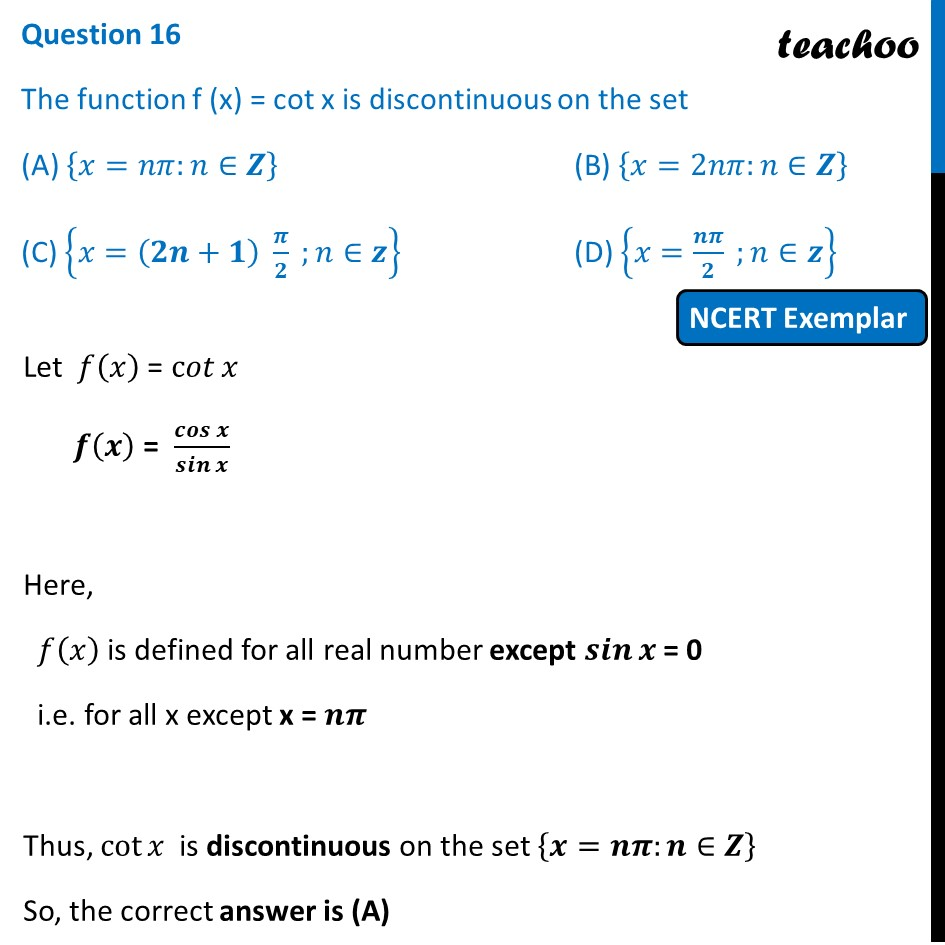NCERT Exemplar - MCQs

Chapter 5 Class 12 Continuity and Differentiability
Serial order wise

## (D) {x= n π/2   ; n ∈z}

This question is similar to Example 18 - Chapter 5 Class 12 - Continuity and DifferentiabilityLearn in your speed, with individual attention - Teachoo Maths 1-on-1 Class

### Transcript

Question 14 The function f (x) = cot x is discontinuous on the set (A) {𝑥=𝑛𝜋:𝑛∈𝒁} (B) {𝑥=2𝑛𝜋:𝑛∈𝒁} (C) {𝑥=(𝟐𝒏+𝟏) 𝝅/𝟐 ;𝑛∈𝒛} (D) {𝑥=𝒏𝝅/𝟐 ;𝑛∈𝒛} Let 𝑓(𝑥) = c𝑜𝑡⁡𝑥 𝒇(𝒙) = 𝒄𝒐𝒔⁡𝒙/𝒔𝒊𝒏⁡𝒙 Here, 𝑓(𝑥) is defined for all real number except 𝒔𝒊𝒏⁡𝒙 = 0 i.e. for all x except x = 𝒏𝝅 Thus, cot⁡𝑥 is discontinuous on the set {𝒙=𝒏𝝅:𝒏∈𝒁} So, the correct answer is (A)